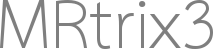Hi,

I want to test my new reconstruction method in SD_Stream tractography. However, only fod can be used in SD_Stream. I have to move my fod from Matlab to Mrtrix tools, steps are as follows.

the dirorder command is used to chage cartesian coordinates direction (642 directions) [x y z] to “[az el]”.
Next, I use amp2sh command with new “[az el]” directions convert my fod files to spherical harmonic coefficient.
Finally, using mrview to check my result.

However, the FOD in mrview isn’t show right directions, and I don’t know what I did wrong.

JZ He

There might be a mismatch between what you consider azimuth and elevation with how mrtrix defines it. Try running it again with the new directions as [el az].

That’s a possible explanation. Another one might be the coordinate frame of reference used: in MRtrix3, these things are expressed in “world” (“scanner”, …) coordinates, not image space (“voxel space”) coordinates.

First try and resolve major flips or 90 degree types of mismatches by permuting or flipping x, y, z coordinates before converting to azimuth and elevation pairs. Then check if a remaining orientation mismatch exists as a (somewhat smaller) angle, and check the image transform in the header against that. The latter (if the case) could reveal a mismatch between image and world/scanner/… space.

Throwing a third possibility into the mix: There can be differences in polar coordinate systems in the second of the two angles, in terms of whether it is defined as an elevation or an inclination.

Hi, thanks for your reply. I have tried flips, 90 degree and flips plus 90 degree. However, none of these results were successful. What can I do to apply my estimate of FOD to SDSTREAM?

In case it helps, here is the code that converts Cartesian to spherical coordinates:

``````      cartesian2spherical (const VectorType1& xyz, VectorType2&& az_el_r)
{
auto r = std::sqrt (Math::pow2(xyz) + Math::pow2(xyz) + Math::pow2(xyz));
az_el_r = std::atan2 (xyz, xyz);
az_el_r = std::acos (xyz / r);
if (az_el_r.size() == 3)
az_el_r = r;
}
``````

Another option would be to modify `amp2sh` to accept Cartesian direction schemes. This is actually trivial to do: open `cmd/amp2sh.cpp`, go to line 207, and modify as follows:

``````  if (opt.size()) {
Then save the file, and type `./build` again. At this point, you should be able to use your Cartesian matrix of directions as-is.